#### Solving a motion planning problem

Cylindrical algebraic decomposition is also capable of solving any of the motion planning problems formulated in Chapter 4. First assume that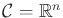. As for other decompositions, a roadmap is formed in which every vertex is an-cell and edges connect every pair of adjacent-cells by traveling through an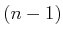-cell. It is straightforward to determine adjacencies inside of a cylinder, but there are several technical details associated with determining adjacencies of cells from different cylinders (pages 152-154 of  present an example that illustrates the problem). The cells of dimension less than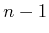are not needed for motion planning purposes (just as vertices were not needed for the vertical decomposition in Section 6.2.2). The query points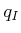and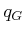are connected to the roadmap depending on the cell in which they lie, and a discrete search is performed.

If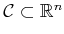and its dimension isfor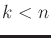, then all of the interesting cells are of lower dimension. This occurs, for example, due to the constraints on the matrices to force them to lie in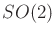or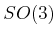. This may also occur for problems from Section 4.4, in which closed chains reduce the degrees of freedom. The cylindrical algebraic decomposition method can still solve such problems; however, the exact root representation problem becomes more complicated when determining the cell adjacencies. A discussion of these issues appears in . For the case ofand, this complication can be avoided by using stereographic projection to map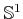or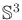toor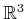, respectively. This mapping removes a single point from each, but the connectivity ofremains unharmed. The antipodal identification problem for unit quaternions represented byalso does not present a problem; there is a redundant copy of, which does not affect the connectivity.

The running time for cylindrical algebraic decomposition depends on many factors, but in general it is polynomial in the number of polynomials in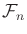, polynomial in the maximum algebraic degree of the polynomials, and doubly exponential in the dimension. Complexity issues are covered in more detail in Section 6.5.3.

Steven M LaValle 2020-08-14